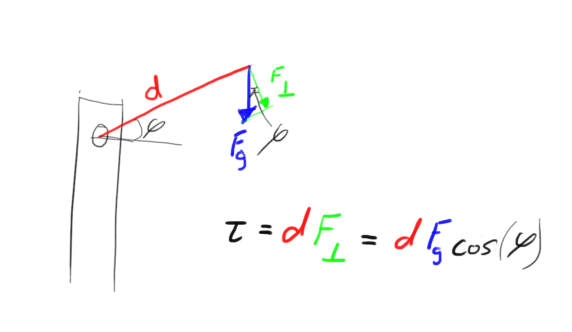# 6-Bar Formula

What is a formula to figure out how tall a 6-Bar can reach? Also, when figuring out how much the 6-Bar can lift, do I measure the longest bar, or the farthest point from the tower?

Just give me as much info/formulas for the 6-BarI don’t know one for height, but for amount to lift:

Torque is (perpendicular force)(distance). For our purposes, ignore the “perpendicular” tag, and just think of torque as (force)(distance). To determine the “worst case” torque on the motors, you want to use the largest force that you’ll ever see (e.g. the weight of the intake + some of the weight of the arm + whatever you’re carrying) placed the furthest distance away from the pivot. So, you’d use the farthest point from the tower.

Note, though, that this is the worst case scenario. That is, this is modelling the robot with it’s arm stretched out as far as it can go. This also doesn’t take into account any elastic. Accounting for elastic is difficult, as you don’t have a spring constant for the tubing, and it’s not really an ideal spring… (or is it? I really don’t know. New summer project!)

To find height, u can add the height of the mass to Xcos(angle it turns). Where X is the length of the center 6 bar.

The formula for torque is correct, however you want to consider that gears have pressure angles. Also to find the torque at specific angles, you need calculus. This is because the distance to center of gravity(radius) will shift. And I do believe springs have the same properties as elastic (force=spring constant X displacement stretched). Becareful with elastic though, this may increase frictional force depending on how you mount it, making it counter productive.

Um… Not to be argumentative, but…For estimation (even at a given angle), you don’t need calculus. The formula you would use is the same, except you have to remember about the perpendicular force part, again:
torque = (distance from pivot) * (Perpendicular force)

A diagram is:The Blue F represents force due to gravity on the arm. The green F is the perpendicular component of the blue F. The symbol tau is for torque. Phi is just whatever angle the arm is at.

When calculus comes in is when you want to take into account the weight of the arm itself, not just the load at the end of the arm.

oh woops, i said specific angle didn’t i…:o because the length between the axle and the end of the arm changes depending on the angle, i immediately thought calculus. But yes, calculating torque using the arm at 90 degrees would be the best estimate to use

+1

Yep this correct. And do be careful with the elastic as it can make the lift perform worse. I would suggest rubber bands instead( if that is not what you meant already) because they provide more force and resistance to ware than the latex tubing in my experience.